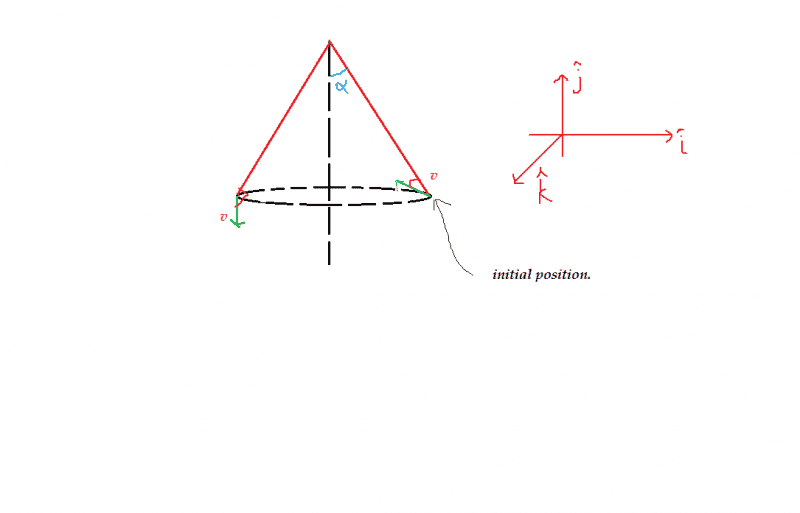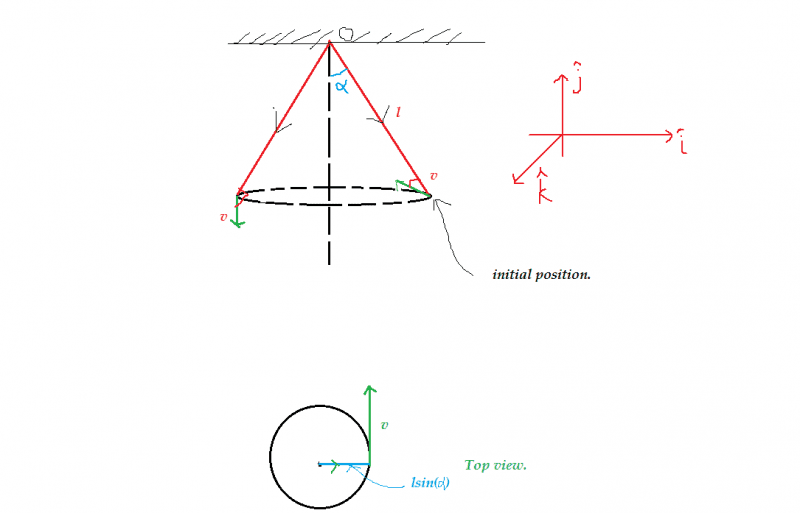# Angular Momentum of a conical pendulum.

## Homework Statement

A small ball of mass m suspended from a ceiling at a point O by a thread of length l moves along a horizontal circle with constant angular velocity ##\omega##. Find the magnitude of increment of the vector of the ball's angular momentum relative to point O picked up during half of revolution.

## The Attempt at a SolutionI[/B]nitial velocity of ball ##V_{i}=v\hat { j }##

Initial distance of the ball from O is (R)=##lsin\alpha\hat{i}-lcos\alpha\hat{k}##

Final velocity ##V_{f}=-V\hat{j}##

Final distance of the ball from O is ##-lsin\alpha\hat{i}-lcos\alpha\hat{k}##

Initial momentum is ##R## cross ##P##.

##L_{i}=mvLcos\alpha\hat{i}-mvlsin\alpha\hat{k}##

##L_{f}=-mvLcos\alpha\hat{i}+mvlsin\alpha\hat{k}##

##\delta L=-2mvLcos\alpha\hat{i}+2mvlsin\alpha\hat{k}##

So its magnitude is ##2mvL##

I got ##cos\alpha=\frac{g}{\omega^2l}## by writing the force equation.

Now ##v=lsin\alpha\omega##

Using this I got the answer

##2ml^{2}\omega\sqrt{1-\frac{g^{2}}{(\omega^{2}l)^2}}##

Can you guys tell me some applications like Daum Equation Editor for writing equations in LaTex. I don't why is it not working in my PC.ehild
Homework Helper
Check your coordinate system and the components of your vectors, they do not matchCheck your coordinate system and the components of your vectors, they do not matchI have considered the circle to be in X-Y plane.I have shown the top view also.

For finding angular momentum of any body about any point(say O) we drop any line from O to the line of the velocity of that body. Then we calculate ##\overrightarrow { R } \times \overrightarrow { p } ##. right?

So ##R_{i}=lsin(\alpha)\hat { i }- lcos(\alpha)\hat { k }##

ehild
Homework Helper
I have considered the circle to be in X-Y plane.View attachment 77622

I have shown the top view also.

For finding angular momentum of any body about any point(say O) we drop any line from O to the line of the velocity of that body. Then we calculate ##\overrightarrow { R } \times \overrightarrow { p } ##. right?

So ##R_{i}=lsin(\alpha)\hat { i }- lcos(\alpha)\hat { k }##

OK, but then the change the top right figure with the unit vectors. And check the signs in Li .
I got

##L_{i}=mvLcos\alpha\hat{i}+mvlsin\alpha\hat{k}##

•Satvik Pandey
OK, but then the change the top right figure with the unit vectors. And check the signs in Li .
I got

##L_{i}=mvLcos\alpha\hat{i}+mvlsin\alpha\hat{k}##

Oh! I made a sign mistake.Now I got answer

##\frac { 2mgl }{ \omega } \sqrt { 1-{ \left( \frac { g }{ { \omega }^{ 2 }l } \right) }^{ 2 } } ##.

Thank you for the help.:)

How is angular momentum conserved from the center of the circle

haruspex